How to enter subscript and superscript values in a cell in Excel [Tip]

If you use Excel spreadsheets to enter chemical equations in, you should add subscript formatting to the formula’s molecules. Subscript formatting is a number, figure or symbol that is smaller than the standard font type. Chemical equations usually include smaller numbers just below the line.

For example, the chemical equation for photosynthesis is 6CO2 + 6H2O ——> C6H12O6 + 6O2. However, that doesn’t include any subscript formatting. You could enter the equation in an Excel spreadsheet without subscript formatting as shown below.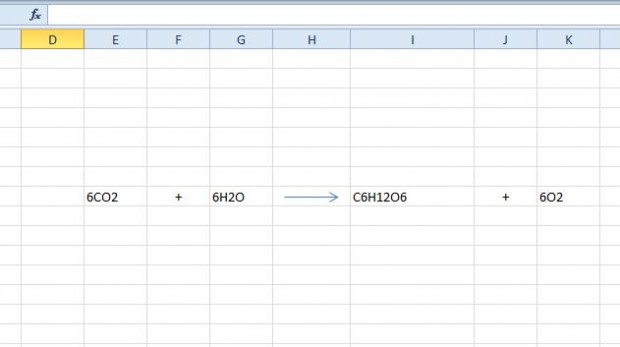To apply the formatting, first select the number in the cell to add the subscript to. So for 6CO2 you would select the 2 in the cell. Then right-click and select Format Cells to open the window in the shot below.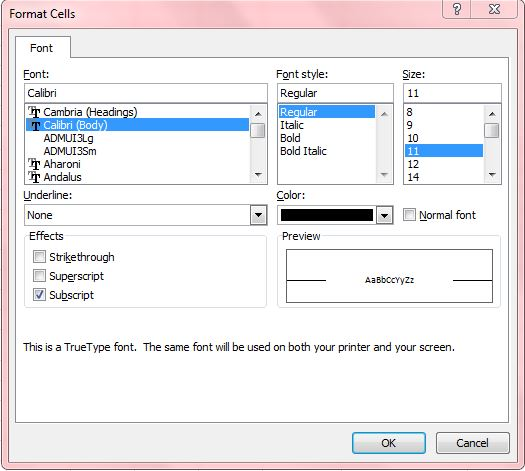In that window select the Subscript option. Then press the OK button to close the window. The number you selected will then have subscript formatting as shown below.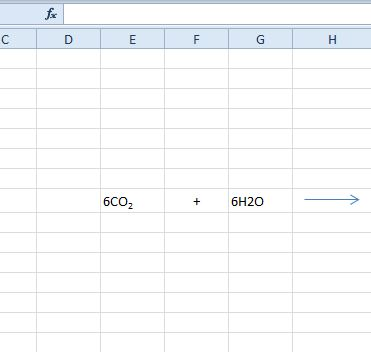Now you can apply the subscript formatting to the rest of the equation much the same. Then it will be more like the one shown the shot below.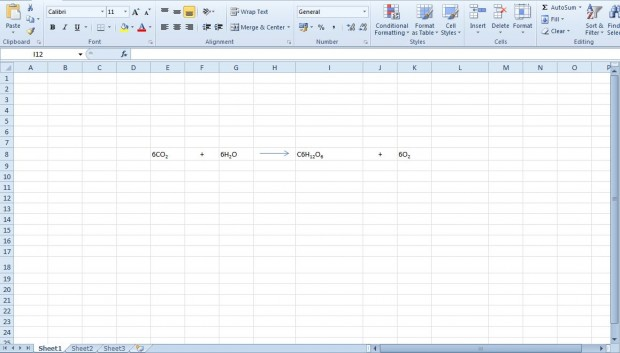Superscripts, smaller numbers just above the line, are also added to atomic isotopes and other chemical symbols. You can select a Superscript option from Excel’s Format Cell window much the same as the subscripts. That adds a superscript to the selected number as shown below.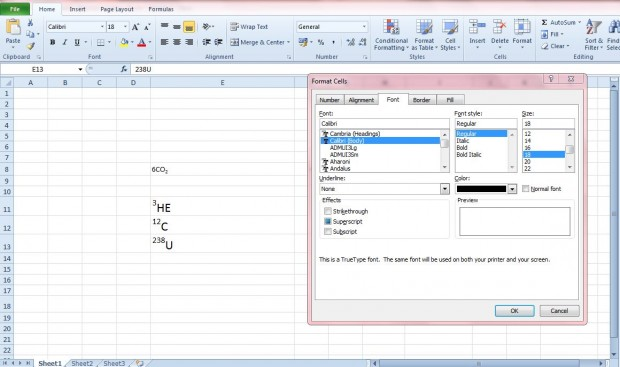Those Excel formatting options will certainly enhance chemistry spreadsheets. With the options you can now add subscripts for molecular compounds and superscripts to atomic isotopes entered into cells. The subscript and superscript formatting options will also be handy for algebra equations.